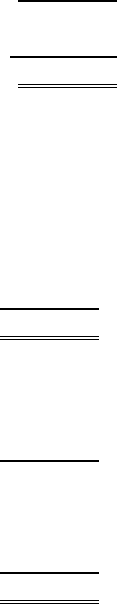# MGAB01H3 Chapter Notes - Chapter 1: Accounts Payable, Issued Shares

40 views5 pages
School
Course
ProfessorE1–3
THE UNIVERSITY SHOP
Statement of Earnings
For the Month of September 2015
Revenue from sales \$120,000
(*)
Expenses:
Cost of sales \$ 40,000
Salaries, rent, supplies, and other expenses 38,000
Utilities 600
Total expenses 78,600
Net earnings for the period \$ 41,400
(*) \$119,000 + \$1,000 = \$120,000.
(Note: income taxes were ignored in this problem.)
E1–6
A Net earnings = \$91,700 – \$76,940 = \$14,760;
Shareholders’ Equity = \$140,200 – \$69,000 = \$71,200.
B Total Revenues = \$74,240 + \$14,740 = \$88,980;
Total Liabilities = \$107,880 – \$79,010 = \$28,870.
C Net earnings (Loss) = \$69,260 – \$76,430 = (\$7,170);
Shareholders’ Equity = \$97,850 – \$69,850 = \$28,000.
D Total Expenses = \$58,680 – \$21,770 = \$36,910;
Total Assets = \$17,890 + \$78,680 = \$96,570.
E Net earnings = \$84,840 – \$78,720 = \$6,120;
Total Assets = \$25,520 + \$79,580 = \$105,100.
Unlock document

This preview shows pages 1-2 of the document.
Unlock all 5 pages and 3 million more documents.E1–7
DUCHARME CORPORATION
Summary Statement of Earnings
For the Month of January 2014
Total revenues \$299,000
Less: Total expenses (excluding income taxes) 189,000
Earnings before income taxes 110,000
Less: Income tax expense 34,500
Net earnings \$ 75,500
DUCHARME CORPORATION
Statement of Financial Position
As at January 31, 2014
Assets
Cash \$ 65,150
Receivables from customers 34,500
Merchandise inventory 96,600
Total assets \$196,250
Liabilities and Shareholders’ Equity
Liabilities:
Payables to suppliers \$ 26,450
Income taxes payable 34,500
Total liabilities 60,950
Shareholders’ equity:
Contributed capital (2,600 shares issued) 59,800
Retained earnings 75,500
Total liabilities and shareholders’ equity \$196,250
Unlock document

This preview shows pages 1-2 of the document.
Unlock all 5 pages and 3 million more documents.

## Document Summary

Net earnings for the period (*) ,000 + ,000 = ,000. (note: income taxes were ignored in this problem. ) Net earnings = ,700 ,940 = ,760; Shareholders" equity = ,200 ,000 = ,200. Total revenues = ,240 + ,740 = ,980; Total liabilities = ,880 ,010 = ,870. Net earnings (loss) = ,260 ,430 = (,170); Shareholders" equity = ,850 ,850 = ,000. Total expenses = ,680 ,770 = ,910; Total assets = ,890 + ,680 = ,570. Net earnings = ,840 ,720 = ,120; Total assets = ,520 + ,580 = ,100. The personal residences of the organizers are not resources of the business entity. It is not indicated whether the ,000 listed for service supplies and the. ,000 listed for service trucks and equipment reflect their cost when acquired or the current market value on december 31, 2016. Furthermore, the amount listed for bills from customers may not represent the potentially collectable amount.

# Get access

\$10 USD/m
Billed \$120 USD annually
Homework Help
Class Notes
Textbook Notes